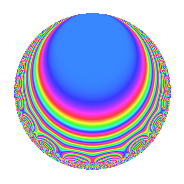# Properties

 Label 50.2.eLevel 50 Weight 2 Character orbit e Rep. character $$\chi_{50}(9,\cdot)$$ Character field $$\Q(\zeta_{10})$$ Dimension 8 Newforms 1 Sturm bound 15 Trace bound 0

# Learn more about

## Defining parameters

 Level: $$N$$ = $$50 = 2 \cdot 5^{2}$$ Weight: $$k$$ = $$2$$ Character orbit: $$[\chi]$$ = 50.e (of order $$10$$ and degree $$4$$) Character conductor: $$\operatorname{cond}(\chi)$$ = $$25$$ Character field: $$\Q(\zeta_{10})$$ Newforms: $$1$$ Sturm bound: $$15$$ Trace bound: $$0$$

## Dimensions

The following table gives the dimensions of various subspaces of $$M_{2}(50, [\chi])$$.

Total New Old
Modular forms 40 8 32
Cusp forms 24 8 16
Eisenstein series 16 0 16

## Trace form

 $$8q$$ $$\mathstrut +\mathstrut 2q^{4}$$ $$\mathstrut -\mathstrut 10q^{5}$$ $$\mathstrut +\mathstrut 2q^{6}$$ $$\mathstrut -\mathstrut 4q^{9}$$ $$\mathstrut +\mathstrut O(q^{10})$$ $$8q$$ $$\mathstrut +\mathstrut 2q^{4}$$ $$\mathstrut -\mathstrut 10q^{5}$$ $$\mathstrut +\mathstrut 2q^{6}$$ $$\mathstrut -\mathstrut 4q^{9}$$ $$\mathstrut -\mathstrut 4q^{11}$$ $$\mathstrut -\mathstrut 10q^{12}$$ $$\mathstrut -\mathstrut 4q^{14}$$ $$\mathstrut -\mathstrut 20q^{15}$$ $$\mathstrut -\mathstrut 2q^{16}$$ $$\mathstrut +\mathstrut 10q^{17}$$ $$\mathstrut +\mathstrut 10q^{19}$$ $$\mathstrut +\mathstrut 16q^{21}$$ $$\mathstrut +\mathstrut 20q^{22}$$ $$\mathstrut +\mathstrut 10q^{23}$$ $$\mathstrut +\mathstrut 8q^{24}$$ $$\mathstrut +\mathstrut 10q^{25}$$ $$\mathstrut -\mathstrut 28q^{26}$$ $$\mathstrut +\mathstrut 10q^{28}$$ $$\mathstrut +\mathstrut 10q^{29}$$ $$\mathstrut +\mathstrut 20q^{30}$$ $$\mathstrut +\mathstrut 6q^{31}$$ $$\mathstrut -\mathstrut 30q^{33}$$ $$\mathstrut -\mathstrut 4q^{34}$$ $$\mathstrut +\mathstrut 10q^{35}$$ $$\mathstrut +\mathstrut 4q^{36}$$ $$\mathstrut -\mathstrut 10q^{37}$$ $$\mathstrut -\mathstrut 8q^{39}$$ $$\mathstrut -\mathstrut 14q^{41}$$ $$\mathstrut -\mathstrut 10q^{42}$$ $$\mathstrut -\mathstrut 6q^{44}$$ $$\mathstrut +\mathstrut 10q^{45}$$ $$\mathstrut -\mathstrut 8q^{46}$$ $$\mathstrut -\mathstrut 30q^{47}$$ $$\mathstrut -\mathstrut 10q^{48}$$ $$\mathstrut -\mathstrut 16q^{49}$$ $$\mathstrut +\mathstrut 16q^{51}$$ $$\mathstrut -\mathstrut 20q^{54}$$ $$\mathstrut -\mathstrut 10q^{55}$$ $$\mathstrut +\mathstrut 4q^{56}$$ $$\mathstrut -\mathstrut 14q^{61}$$ $$\mathstrut +\mathstrut 20q^{63}$$ $$\mathstrut +\mathstrut 2q^{64}$$ $$\mathstrut +\mathstrut 50q^{65}$$ $$\mathstrut +\mathstrut 24q^{66}$$ $$\mathstrut +\mathstrut 10q^{67}$$ $$\mathstrut -\mathstrut 8q^{69}$$ $$\mathstrut -\mathstrut 34q^{71}$$ $$\mathstrut +\mathstrut 36q^{74}$$ $$\mathstrut +\mathstrut 10q^{75}$$ $$\mathstrut +\mathstrut 40q^{77}$$ $$\mathstrut +\mathstrut 20q^{78}$$ $$\mathstrut -\mathstrut 12q^{81}$$ $$\mathstrut +\mathstrut 50q^{83}$$ $$\mathstrut +\mathstrut 14q^{84}$$ $$\mathstrut -\mathstrut 20q^{85}$$ $$\mathstrut +\mathstrut 22q^{86}$$ $$\mathstrut +\mathstrut 20q^{87}$$ $$\mathstrut -\mathstrut 10q^{88}$$ $$\mathstrut +\mathstrut 20q^{89}$$ $$\mathstrut -\mathstrut 30q^{90}$$ $$\mathstrut -\mathstrut 4q^{91}$$ $$\mathstrut -\mathstrut 10q^{92}$$ $$\mathstrut -\mathstrut 24q^{94}$$ $$\mathstrut +\mathstrut 2q^{96}$$ $$\mathstrut -\mathstrut 20q^{97}$$ $$\mathstrut -\mathstrut 40q^{98}$$ $$\mathstrut -\mathstrut 28q^{99}$$ $$\mathstrut +\mathstrut O(q^{100})$$

## Decomposition of $$S_{2}^{\mathrm{new}}(50, [\chi])$$ into irreducible Hecke orbits

Label Dim. $$A$$ Field CM Traces $q$-expansion
$$a_2$$ $$a_3$$ $$a_5$$ $$a_7$$
50.2.e.a $$8$$ $$0.399$$ $$\Q(\zeta_{20})$$ None $$0$$ $$0$$ $$-10$$ $$0$$ $$q+\zeta_{20}q^{2}+(-1+\zeta_{20}^{2}-\zeta_{20}^{4}+2\zeta_{20}^{6}+\cdots)q^{3}+\cdots$$

## Decomposition of $$S_{2}^{\mathrm{old}}(50, [\chi])$$ into lower level spaces

$$S_{2}^{\mathrm{old}}(50, [\chi]) \cong$$ $$S_{2}^{\mathrm{new}}(25, [\chi])$$$$^{\oplus 2}$$